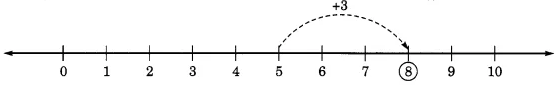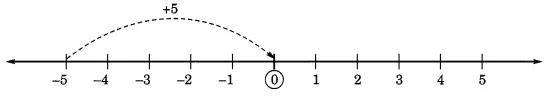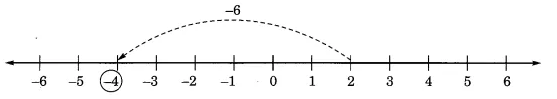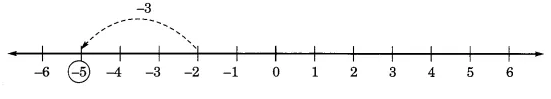# 1.  Using the number line write the integer which is :   (a) more than     (b) more than    (c) less than    (d)  less than

M manish painkra

(a) more than
The answer is 5 + 3  = 8(b) more than
The answer is 5 + (-5) = 0(c) less than
the answer is 2 - 6 = -4(d)  less than
The answer is 3 + (-2) = 1Exams
Articles
Questions